Skip to main content

# A Study on the-Adic Integral Representation onAssociated with Bernstein and Bernoulli Polynomials

## Abstract

We consider the Bernstein polynomials onand investigate some interesting properties of Bernstein polynomials related to Stirling numbers and Bernoulli numbers.

## 1. Introduction

Letdenote the set of continuous function on. Then, Bernstein operator foris defined as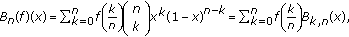(1.1)

for, where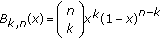is called Bernstein polynomial of degree. Some researchers have studied the Bernstein polynomials in the area of approximation theory (see ).

Letbe a fixed prime number. Throughout this paper,,, andwill, respectively, denote the ring of-adic rational integers, the field of-adic rational numbers, the complex number field, and the completion of algebraic closure of. Letbe the set of uniformly differentiable function on. For, the-adic-integral onis defined by(1.2)

(see [4, 715]).

In the special case, if we setin (1.2), we have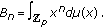(1.3)

In this paper, we consider Bernstein polynomials onand we investigate some interesting properties of Bernstein polynomials related to Stirling numbers and Bernoulli numbers.

## 2. Bernstein Polynomials Related to Stirling Numbers and Bernoulli Numbers

In this section, for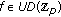, we consider Bernstein type operator onas follows: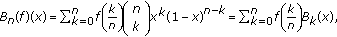(2.1)

for, whereis called Bernstein polynomial of degree. We consider Newton's forward difference operator as follows: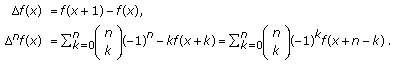(2.2)

For,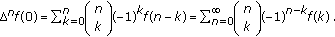(2.3)

Then, we have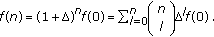(2.4)

From (2.4), we note that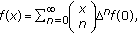(2.5)

where(2.6)

The Stirling number of the first kind is defined by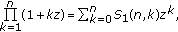(2.7)

and the Stirling number of the second kind is also defined by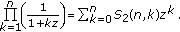(2.8)

By (2.5), (2.6), (2.7), and (2.8), we see that(2.9)

where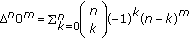. Note that, forand,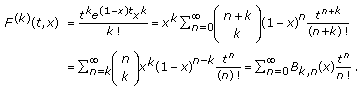(2.10)

Thus, we note thatis the generating function of Bernstein polynomial. It is easy to show that(2.11)

By (2.11), we obtain the following theorem.

Theorem 2.1.

Forwith, one has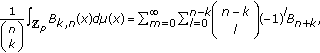(2.12)

whereare theth Bernoulli numbers.

In , it is known that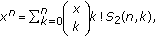(2.13)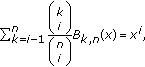(2.14)

for. By (1.1) and (2.14), we see that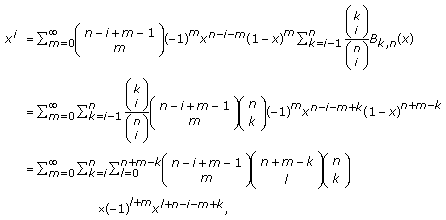(2.15)

for. By (2.15), we obtain the following theorem.

Theorem 2.2.

For, and, one has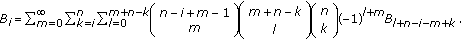(2.16)

From (2.13) and (2.14), we note that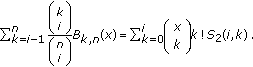(2.17)

In , it is known that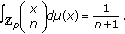(2.18)

By (2.17), (2.18), and Theorem 2.2, we have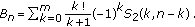(2.19)

From the definition of the Stirling numbers of the first kind, we drive that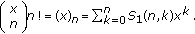(2.20)

By (2.17), (2.19), and (2.20), we obtain the following theorem.

Theorem 2.3.

Forand, one has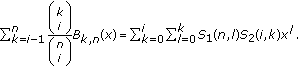(2.21)

By Theorems 2.2 and 2.3, we obtain the following corollary.

Corollary 2.4.

For, one has(2.22)

whereare theth Bernoulli numbers.

## References

1. 1.

Acikgoz M, Araci S: A study on the integral of the product of several type Bernstein polynomials. IST Transaction of Applied Mathematics-Modelling and Simulation. In press

2. 2.

Acikgoz M, Araci S: On the generating function of the Bernstein polynomials. In Proceedings of the 8th International Conference of Numerical Analysis and Applied Mathematics (ICNAAM '10), March 2010, Rhodes, Greece. AIP;

3. 3.

Bernstein S: Demonstration du theoreme de Weierstrass, fondee sur le calcul des probabilities. Communications of the Kharkov Mathematical Society 1913, 13: 1-2.

4. 4.

Kim T, Jang LC, Yi H: A note on the modified q -Bernstein polynomials. Discrete Dynamics in Nature and Society 2010, 2010:-12.

5. 5.

Phillips GM: Bernstein polynomials based on the q -integers. Annals of Numerical Mathematics 1997,4(1–4):511-518.

6. 6.

Kim MS, Kim D, Kim T: On the q-Euler numbers related to modified q-Bernstein polynomials. http://arxiv.org/abs/1007.3317

7. 7.

Kim T: q -Volkenborn integration. Russian Journal of Mathematical Physics 2002,9(3):288-299.

8. 8.

Kim T: q -Bernoulli numbers and polynomials associated with Gaussian binomial coefficients. Russian Journal of Mathematical Physics 2008,15(1):51-57.

9. 9.

Kim T: Note on the Euler q -zeta functions. Journal of Number Theory 2009,129(7):1798-1804. 10.1016/j.jnt.2008.10.007

10. 10.

Kim T: On a q -analogue of the p -adic log gamma functions and related integrals. Journal of Number Theory 1999,76(2):320-329. 10.1006/jnth.1999.2373

11. 11.

Kim T: Barnes-type multiple q -zeta functions and q -Euler polynomials. Journal of Physics A 2010.,43(25):

12. 12.

Kim T: Power series and asymptotic series associated with the q -analog of the two-variable p -adic L -function. Russian Journal of Mathematical Physics 2005,12(2):186-196.

13. 13.

Kim T, Choi J, Kim Y-H: Some identities on the q-Bernstein polynomials, g-Stirling numbers and q-Bernoulli numbers. Advanced Studies in Contemporary Mathematics 2010,20(3):335-341.

14. 14.

Kim T: Some identities on the q -Euler polynomials of higher order and q -Stirling numbers by the fermionic p -adic integral on. Russian Journal of Mathematical Physics 2009,16(4):484-491. 10.1134/S1061920809040037

15. 15.

Kim T: On p -adic interpolating function for q -Euler numbers and its derivatives. Journal of Mathematical Analysis and Applications 2008,339(1):598-608. 10.1016/j.jmaa.2007.07.027

16. 16.

Kim T, Park D-W, Rim S-H: On multivariate p -adic q -integrals. Journal of Physics A 2001,34(37):7633-7638. 10.1088/0305-4470/34/37/315

Download references

## Acknowledgment

The present investigation was supported by the Scientific Research Project Administration of Akdeniz University.

## Author information

Authors

### Corresponding author

Correspondence to Lee-Chae Jang.

## Rights and permissions

Open Access This article is distributed under the terms of the Creative Commons Attribution 2.0 International License (https://creativecommons.org/licenses/by/2.0), which permits unrestricted use, distribution, and reproduction in any medium, provided the original work is properly cited.

Reprints and Permissions

## About this article

### Cite this article

Jang, LC., Kim, WJ. & Simsek, Y. A Study on the-Adic Integral Representation onAssociated with Bernstein and Bernoulli Polynomials. Adv Differ Equ 2010, 163217 (2010). https://doi.org/10.1155/2010/163217

Download citation

• Received:

• Accepted:

• Published:

### Keywords

• Ordinary Differential Equation
• Functional Equation
• Prime Number
• Rational Number
• Difference Operator